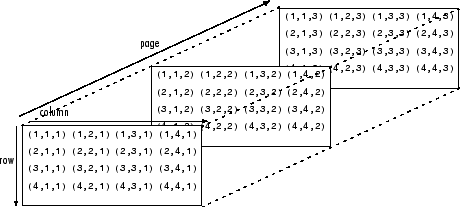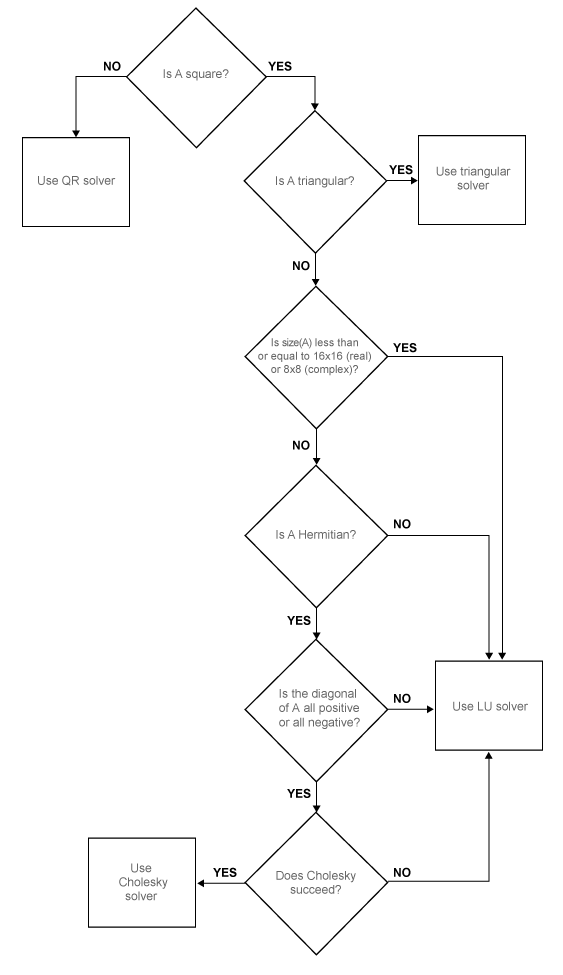# pagemldivide

Page-wise left matrix divide

Since R2022a

## Syntax

``X = pagemldivide(A,B)``
``X = pagemldivide(A,transpA,B)``
``[X,rcondA] = pagemldivide(___)``

## Description

example

````X = pagemldivide(A,B)` computes the left matrix divide of each page of N-D array `A` into each page of N-D array `B`. Each page of the output array `X` is given by `X(:,:,i) = A(:,:,i) \ B(:,:,i)`. The pages of `A` and `B` must be valid inputs to `mldivide` (`\`).If `A` and `B` have more than three dimensions, then all dimensions beyond the first two must have compatible sizes. `pagemldivide` implicitly expands the extra dimensions to divide all page combinations: ```X(:,:,i,j,k) = A(:,:,i,j,k) \ B(:,:,i,j,k)```. ```

example

````X = pagemldivide(A,transpA,B)` optionally applies a transposition to each page of `A`. The value of `transpA` can be `"transpose"`, `"ctranspose"`, or `"none"`. For example, `pagemldivide(A,"transpose",B)` computes ```X(:,:,i) = A(:,:,i).' \ B(:,:,i)```.```

example

````[X,rcondA] = pagemldivide(___)` also returns the reciprocal condition number of each page of `A`, using any of the input argument combinations in previous syntaxes. The value of `rcondA(1,1,i)` is the reciprocal condition number of `A(:,:,i)`. If `rcondA(1,1,i) < eps`, then `X(:,:,i) = A(:,:,i) \ B(:,:,i)` returns a warning because the matrix is ill conditioned. However, `pagemldivide` does not issue a warning for ill-conditioned inputs.```

## Examples

collapse all

Create a 3-by-3-by-2 array `A` that has 3-by-3 matrices on each of two pages. Also create a 3-by-1-by-2 array `B`.

```p1 = magic(3); p2 = hilb(3); A = cat(3,p1,p2)```
```A = A(:,:,1) = 8 1 6 3 5 7 4 9 2 A(:,:,2) = 1.0000 0.5000 0.3333 0.5000 0.3333 0.2500 0.3333 0.2500 0.2000 ```
`B = ones(3,1,2)`
```B = B(:,:,1) = 1 1 1 B(:,:,2) = 1 1 1 ```

Solve the equation `A(:,:,i)*X(:,:,i) = B(:,:,i)` for each corresponding set of pages in `A` and `B`.

`X = pagemldivide(A,B)`
```X = X(:,:,1) = 0.0667 0.0667 0.0667 X(:,:,2) = 3.0000 -24.0000 30.0000 ```

Create a 4-by-4-by-2 array `A` that has 4-by-4 matrices on each of two pages. Also create a 4-by-1-by-2 array `B`.

```p1 = pascal(4); p2 = magic(4); A = cat(3,p1,p2)```
```A = A(:,:,1) = 1 1 1 1 1 2 3 4 1 3 6 10 1 4 10 20 A(:,:,2) = 16 2 3 13 5 11 10 8 9 7 6 12 4 14 15 1 ```
`B = 2*ones(4,1,2)`
```B = B(:,:,1) = 2 2 2 2 B(:,:,2) = 2 2 2 2 ```

Specify the `"transpose"` option to solve the system `A(:,:,i).'*X(:,:,i) = B(:,:,i)` for each corresponding set of pages in `A` and `B`.

`X = pagemldivide(A,"transpose",B)`
```X = X(:,:,1) = 2 0 0 0 X(:,:,2) = 0.0263 -0.0386 0.1562 0.0913 ```

Create a 10-by-10-by-2 array `A` that has 10-by-10 matrices on each of two pages. Also create a 10-by-1-by-2 array `B`.

```p1 = diag([ones(9,1);0]); p2 = pascal(10); A = cat(3,p1,p2)```
```A = A(:,:,1) = 1 0 0 0 0 0 0 0 0 0 0 1 0 0 0 0 0 0 0 0 0 0 1 0 0 0 0 0 0 0 0 0 0 1 0 0 0 0 0 0 0 0 0 0 1 0 0 0 0 0 0 0 0 0 0 1 0 0 0 0 0 0 0 0 0 0 1 0 0 0 0 0 0 0 0 0 0 1 0 0 0 0 0 0 0 0 0 0 1 0 0 0 0 0 0 0 0 0 0 0 A(:,:,2) = 1 1 1 1 1 1 1 1 1 1 1 2 3 4 5 6 7 8 9 10 1 3 6 10 15 21 28 36 45 55 1 4 10 20 35 56 84 120 165 220 1 5 15 35 70 126 210 330 495 715 1 6 21 56 126 252 462 792 1287 2002 1 7 28 84 210 462 924 1716 3003 5005 1 8 36 120 330 792 1716 3432 6435 11440 1 9 45 165 495 1287 3003 6435 12870 24310 1 10 55 220 715 2002 5005 11440 24310 48620 ```
`B = 3*ones(10,1,2)`
```B = B(:,:,1) = 3 3 3 3 3 3 3 3 3 3 B(:,:,2) = 3 3 3 3 3 3 3 3 3 3 ```

Solve the equation `A(:,:,i)*X(:,:,i) = B(:,:,i)` for each corresponding set of pages in `A` and `B`. Specify two outputs to also return the reciprocal condition number of each page in `A`.

`[X,rcondA] = pagemldivide(A,B)`
```X = X(:,:,1) = 3 3 3 3 3 3 3 3 3 Inf X(:,:,2) = 3 0 0 0 0 0 0 0 0 0 ```
```rcondA = rcondA(:,:,1) = 0 rcondA(:,:,2) = 1.2295e-10 ```

Compare the reciprocal condition numbers to `eps`. The results indicate that the matrix on the first page is ill conditioned, so the command `A(:,:,1)\B(:,:,1)` returns a warning and the results of the operation for that page are not reliable.

`rcondA < eps`
```ans = 1x1x2 logical array ans(:,:,1) = 1 ans(:,:,2) = 0 ```

## Input Arguments

collapse all

Input arrays. `A` and `B` are multidimensional arrays where each corresponding set of pages `A(:,:,i,...)` and `B(:,:,i,...)` are valid inputs to `mldivide`.

If `A` and `B` have more than three dimensions, then all dimensions beyond the first two must have compatible sizes. `pagemldivide` implicitly expands the extra dimensions to divide all page combinations: ```X(:,:,i,j,k) = A(:,:,i,j,k) \ B(:,:,i,j,k)```.

Data Types: `single` | `double`
Complex Number Support: Yes

Transpose option for `A`, specified as one of these values.

ValueDescription
`"none"`Do not transpose `A`. This is the default value.
`"transpose"`Transpose each page of `A` without complex conjugation.
`"ctranspose"`Transpose each page of `A` with complex conjugation.

Example: `pagemldivide(A,"transpose",B)`

## Output Arguments

collapse all

Linear system solutions, returned as a multidimensional array with the same number of pages as `A` and `B`. Each page of `X` is the solution to the linear system ```X(:,:,i,...) = A(:,:,i,...) \ B(:,:,i,...)```.

Reciprocal condition numbers of `A`, returned as a multidimensional array. If the pages of `A` are `m`-by-`n` rectangular matrices with `m~=n`, then `rcondA` is estimated using the entries of `R` in the QR decomposition. If ```rcondA(1,1,i) < eps```, then `X(:,:,i) = A(:,:,i) \ B(:,:,i)` returns a warning because the matrix is ill conditioned. However, `pagemldivide` does not issue a warning for ill-conditioned inputs.

collapse all

### Array Pages

Page-wise functions like `pagemldivide` operate on 2-D matrices that have been arranged into a multidimensional array. For example, with a 3-D array the elements in the third dimension of the array are commonly called pages because they stack on top of each other like pages in a book. Each page is a matrix that gets operated on by the function.You can also assemble a collection of 2-D matrices into a higher dimensional array, like a 4-D or 5-D array, and in these cases `pagemldivide` still treats the fundamental unit of the array as a 2-D matrix that gets operated on, such as `X(:,:,i,j,k,l)`.

The `cat` function is useful for assembling a collection of matrices into a multidimensional array, and the `zeros` function is useful for preallocating a multidimensional array.

## Tips

• Results obtained using `pagemldivide` are numerically equivalent to computing the linear system solutions with each of the same matrices in a `for`-loop. However, the two results might differ slightly due to floating-point round-off error.

## Algorithms

Similar to `mldivide`, the `pagemldivide` function determines which algorithm to use in solving the linear systems in the input array by applying a series of checks to the array pages. Different algorithms can be used with different pages in the input array. `pagemldivide` chooses between QR, Triangular, LU, and Cholesky solvers for each array page in `A` depending on its properties, as shown in this diagram.## Version History

Introduced in R2022a

expand all